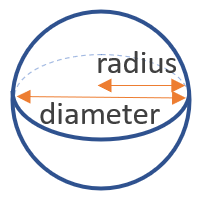# Volume of a Sphere Calculator

Use this sphere volume calculator to easily calculate the volume of a sphere, given its radius in any metric: mm, cm, meters, km, inches, feet, yards, miles, etc.

### Calculation results

113.097336 cm3 Sphere volume
Share calculator:

Embed this tool:
get code

## Volume of a sphere formula

The volume of a sphere is 4/3 x π x (diameter / 2)3, where (diameter / 2) is the radius of the sphere (d = 2 x r), so another way to write it is 4/3 x π x radius3. Visual on the figure below:Since in most practical situations you know the diameter (via measurement or from a plan/schematic), the first formula is usually most useful, but it's easy to do it both ways. The unit of the resulting number is the cube of the length unit used for the radius/diameter. So the result from our volume of a sphere calculator is in cubic inches, cubic feet, cubic yards, cubic miles, or in the metric system: cubic cm, cubic meters, etc.

## How to calculate the volume of a sphere?

Usually the hardest part is measuring or estimating the diameter of the sphere. Special tools exist for smaller parts like balls in ball-bearings, but it gets more complicated if the size is large. Knowing that the diameter is the largest internal measurement you can take should help.

Once you have the measurement, to find the volume use the formula above, in which π is the well-known mathematical constant equal to about 3.14159. To adjust for a half-sphere calculation, just divide the result by two.

Spheres and half-spheres are useful in engineering and architecture due to their property of being able to take equal amounts of pressure or force from each direction.

## Example: find the volume of a sphere

Only a single measurement needs to be known in order to compute the volume of a sphere and that is its diameter. For example, if the diameter is known to be 20 feet, then calculate the volume by using the first formula above to get 4/3 x 3.14159 x (20/2)3 = 4.1866 x 1000 = 4188.79 ft3 (cubic feet).

Alternatively, if the radius is given, multiply it by two to get the diameter or directly use the second equation provided above instead. For example, if the radius of a sphere is 5 inches, using the second sphere volume equation results in 4/3 x 3.14159 x 53 = 4.1866 x 125 = 523.6 in3 (cubic inches).

#### Cite this calculator & page

If you'd like to cite this online calculator resource and information as provided on the page, you can use the following citation:
Georgiev G.Z., "Volume of a Sphere Calculator", [online] Available at: https://www.gigacalculator.com/calculators/volume-of-sphere-calculator.php URL [Accessed Date: 28 Mar, 2023].